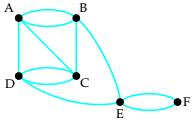# (a) determine whether the graph is Eulerian. If it is, find an Euler circuit. If it is not, explain why. (b)If the graph does not have an Euler circuit, does it have an Euler path? If so, find one. If not, explain why.### Mathematical Excursions (MindTap C...

4th Edition
Richard N. Aufmann + 3 others
Publisher: Cengage Learning
ISBN: 9781305965584

#### Solutions

Chapter
Section### Mathematical Excursions (MindTap C...

4th Edition
Richard N. Aufmann + 3 others
Publisher: Cengage Learning
ISBN: 9781305965584
Chapter 5.1, Problem 20ES
Textbook Problem
15 views

## (a) determine whether the graph is Eulerian. If it is, find an Euler circuit. If it is not, explain why. (b)If the graph does not have an Euler circuit, does it have an Euler path? If so, find one. If not, explain why.To determine

To determine if the graph is Eulerian. If it is, to find an Euler circuit else to explain why not. And if the graph does not have an Euler circuit, to check if it has an Euler path. If it has to find it else to explain the reason for not having an Euler path.

### Explanation of Solution

Given information:

Given graph is

Calculation:

A graph is said to be Eulerian graph if it is a connected graph and every vertex of the graph has even degree.

Here,

The degree of A is 4

The degree of B is 4

The degree of C is 4

The degree of D is 4

The degree of E is 4

The degree of F is 2

Since the graph is connected and all the vertices have even degree, the graph is Eulerian...

### Still sussing out bartleby?

Check out a sample textbook solution.

See a sample solution

#### The Solution to Your Study Problems

Bartleby provides explanations to thousands of textbook problems written by our experts, many with advanced degrees!

Get Started

Find more solutions based on key concepts
In problems 37-48, compute and simplify so that only positive exponents remain. 44.

Mathematical Applications for the Management, Life, and Social Sciences

Evaluate the expression sin Exercises 116. (14)2

Finite Mathematics and Applied Calculus (MindTap Course List)

Find the distance between the points. 1. (1, 1), (4, 5)

Single Variable Calculus: Early Transcendentals, Volume I

In Exercises 1-8, use the graph of the given function f to determine limxaf(x) at the indicated value of a, if ...

Applied Calculus for the Managerial, Life, and Social Sciences: A Brief Approach

Evaluate the indefinite integral. cotxdx

Single Variable Calculus: Early Transcendentals

Given: ABCDBADCDA Prove: AED is isosceles HINT: Prove

Elementary Geometry for College Students

Estimating Rates of Change By direct calculation, estimate the value of dfdx for f(x)=x2+1 at x=3. Use an incre...

Functions and Change: A Modeling Approach to College Algebra (MindTap Course List)

How much work is done in lifting a 60-pound bag of bird seed 3 feet in the air? 20 ft-lbs 20g ft-lbs 180 ft-lbs...

Study Guide for Stewart's Single Variable Calculus: Early Transcendentals, 8th

The graph of x = cos t, y = sin2 t is:

Study Guide for Stewart's Multivariable Calculus, 8th